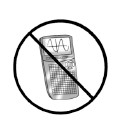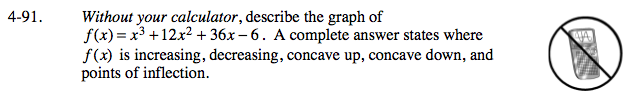### Home > CALC > Chapter 4 > Lesson 4.2.4 > Problem4-91

4-91.

Without your calculator, describe the graph of f(x) = x3 + 12x2 + 36x − 6. A complete answer states where f(x) is increasing, decreasing, concave up, concave down, and points of inflection. Homework Help ✎When f '(x) > 0, f(x) is increasing. When f '(x) < 0, f(x) is decreasing. An inflection point occurs when f '(x) = 0. When f ''(x) > 0, the function is concave up. When f ''(x) < 0, the function is concave down.